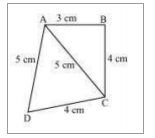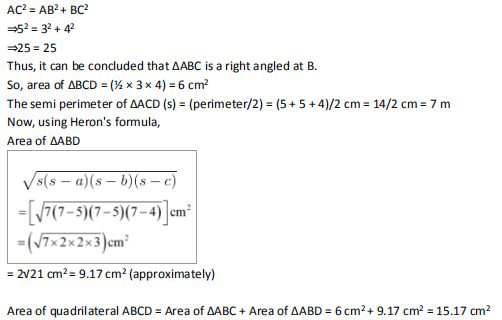# NCERT Solutions Class 9 Mathematics Solutions for Heron’s Formula - Exercise 12.2 in Chapter 12 - Heron’s Formula

Find the area of a quadrilateral ABCD in which AB = 3 cm, BC = 4 cm, CD = 4 cm, DA = 5 cm

and AC = 5 cm.

First, construct a diagram with the given parameterNow, apply the Pythagorean theorem in ΔABCVideo transcript
Related Questions

Lido

Courses

Teachers

Book a Demo with us

Syllabus

Maths
CBSE
Maths
ICSE
Science
CBSE

Science
ICSE
English
CBSE
English
ICSE
Coding

Terms & Policies

Selina Question Bank

Maths
Physics
Biology

Allied Question Bank

Chemistry
Connect with us on social media!